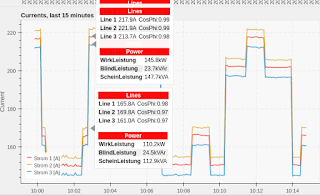# Bokeh: Single tooltip for multiple plots(Also published in stackoverflow: https://stackoverflow.com/questions/46035469/bokeh-single-tooltip-for-multiple-plots)
3 plots on a graph, I want to have a summary tooltip.
When the mouse pointer is close to the 3 graphs I see multiple overlapping tooltips, and this is not nice as they show the same info.
I would like to show this summary by a single tooltip whatever plot is touched by the mouse pointer.
At the moment I can apply a tooltip to a single plot by means of names, but nothing is of course shown when touching the other two.
Is there any way to base the tooltip on the vertical position instead of on a plot? I mean, show the tooltip as soon as the mouse enter the graph even without touching any plot according to its horizontal position.

This is a snippet of my code:
COMMON_PARAM = dict(x=“date_time”, source=self.data_source, line_alpha=GRAPH_LINE_ALPHA, line_width=GRAPH_LINE_WIDTH) line1 = self.figure.line(y=f1, line_color=GRAPH_LINE_1_COLOR, name=“line_with_hovertool”, **COMMON_PARAM) line2 = self.figure.line(y=f2, line_color=GRAPH_LINE_2_COLOR, **COMMON_PARAM) line3 = self.figure.line(y=f3), line_color=GRAPH_LINE_3_COLOR, **COMMON_PARAM) hover = HoverTool( names=[“line_with_hovertool”], # applies only to line1 tooltips= “”" … “”") self.figure.add_tools(hover)

``````
``````

Code in the original post is unreadable, here it is again:

``````
COMMON_PARAM = dict(x="date_time", source=self.data_source, line_alpha=GRAPH_LINE_ALPHA, line_width=GRAPH_LINE_WIDTH)
line1 = self.figure.line(y=f1,
line_color=GRAPH_LINE_1_COLOR, name="line_with_hovertool",
**COMMON_PARAM)
line2 = self.figure.line(y=f2,
line_color=GRAPH_LINE_2_COLOR,
**COMMON_PARAM)
line3 = self.figure.line(y=f3),
line_color=GRAPH_LINE_3_COLOR,
**COMMON_PARAM)
hover = HoverTool(
names=["line_with_hovertool"], # applies only to line1
tooltips=
"""
....
""")